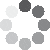Enter parameters Rectangle
Enter parameters
Length:
ft
• inches (in)
• feet (ft)
• yards (yd)
• centimeters (cm)
• meters (m)
Depth:
ft
• inches (in)
• feet (ft)
• yards (yd)
• centimeters (cm)
• meters (m)
Width:
ft
• inches (in)
• feet (ft)
• yards (yd)
• centimeters (cm)
• meters (m)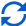Result
Volume:
0
US gal
• US Gallons (US gal)
• UK Gallons (UK gal)
• Liters (l)
Surface area:
0
ft²
• square feet (ft²)
• square inches (in²)
• square yards (yd²)
• square miles (mi²)
• acres (ac)
• square centimeters (cm²)
• square meters ()
Liner Size:
0
ft
• inches (in)
• feet (ft)
• yards (yd)
• centimeters (cm)
• meters (m)
Pump Size:
0
US GPH
• US Gallons Per Hour (US GPH)
• UK Gallons Per Hour (UK GPH)
• Liters Per Hour (LPH)
Enter parameters
Maximum Length:
ft
• inches (in)
• feet (ft)
• yards (yd)
• centimeters (cm)
• meters (m)
Maximum Depth:
ft
• inches (in)
• feet (ft)
• yards (yd)
• centimeters (cm)
• meters (m)
Maximum Width:
ft
• inches (in)
• feet (ft)
• yards (yd)
• centimeters (cm)
• meters (m)Result (approximate)
Volume:
0
US gal
• US Gallons (US gal)
• UK Gallons (UK gal)
• Liters (l)
Surface area:
0
ft²
• square feet (ft²)
• square inches (in²)
• square yards (yd²)
• square miles (mi²)
• acres (ac)
• square centimeters (cm²)
• square meters ()
Liner Size:
0
ft
• inches (in)
• feet (ft)
• yards (yd)
• centimeters (cm)
• meters (m)
Pump Size:
0
US GPH
• US Gallons Per Hour (US GPH)
• UK Gallons Per Hour (UK GPH)
• Liters Per Hour (LPH)
Enter parameters
Diameter:
ft
• inches (in)
• feet (ft)
• yards (yd)
• centimeters (cm)
• meters (m)
Depth:
ft
• inches (in)
• feet (ft)
• yards (yd)
• centimeters (cm)
• meters (m)Result
Volume:
0
US gal
• US Gallons (US gal)
• UK Gallons (UK gal)
• Liters (l)
Surface area:
0
ft²
• square feet (ft²)
• square inches (in²)
• square yards (yd²)
• square miles (mi²)
• acres (ac)
• square centimeters (cm²)
• square meters ()
Liner Size:
0
ft
• inches (in)
• feet (ft)
• yards (yd)
• centimeters (cm)
• meters (m)
Pump Size:
0
US GPH
• US Gallons Per Hour (US GPH)
• UK Gallons Per Hour (UK GPH)
• Liters Per Hour (LPH)
Enter parameters
Length:
ft
• inches (in)
• feet (ft)
• yards (yd)
• centimeters (cm)
• meters (m)
Depth:
ft
• inches (in)
• feet (ft)
• yards (yd)
• centimeters (cm)
• meters (m)
Width:
ft
• inches (in)
• feet (ft)
• yards (yd)
• centimeters (cm)
• meters (m)Result
Volume:
0
US gal
• US Gallons (US gal)
• UK Gallons (UK gal)
• Liters (l)
Surface area:
0
ft²
• square feet (ft²)
• square inches (in²)
• square yards (yd²)
• square miles (mi²)
• acres (ac)
• square centimeters (cm²)
• square meters ()
Liner Size:
0
ft
• inches (in)
• feet (ft)
• yards (yd)
• centimeters (cm)
• meters (m)
Pump Size:
0
US GPH
• US Gallons Per Hour (US GPH)
• UK Gallons Per Hour (UK GPH)
• Liters Per Hour (LPH)(3 votes, average: 5.00 out of 5)Loading...
Similar Calculators:
Real-time graphics. Make the calculations and see the changes.
Real-time graphics. Make the calculations and see the changes.
Real-time graphics. Make the calculations and see the changes.
Real-time graphics. Make the calculations and see the changes.
Embed
Similar Calculators:You’ll likely agree with us when we say that trying to estimate how much water your pond can hold is very difficult.

Use our pond volume calculator to help! 👍

We will also explain the Mathematics the calculator uses to estimate out how much water your pond can hold.

Contents:

## Pond volume calculator formula

To calculate the total volume of your pond, you must first measure the dimensions of your pool.

These can be in metric units (centimeters or meters) or imperial units (yards, feet or inches).

The calculator then works out the total volume of the pond using the following formulae:

### Rectangular Pond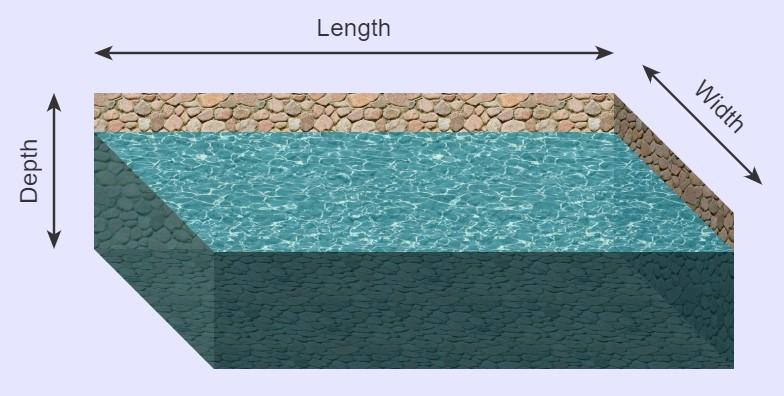For a rectangular pond, the surface area is calculated by:

$$Surface\,Area = Length × Width$$

and the total volume is calculated by:

$$Volume = Surface\,Area × Depth$$

### Irregular Pond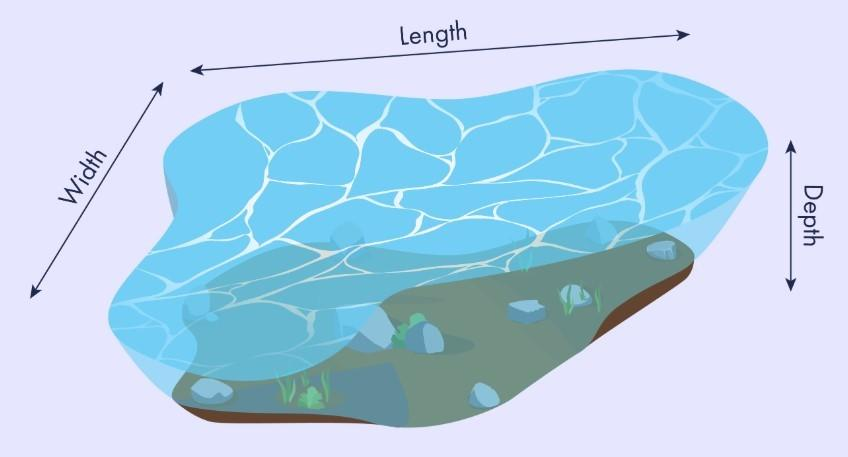As a general rule, the total volume of an irregular pond is a third smaller than a rectangular pond.

We therefore take the volume of the rectangular pond:

$$Volume = Length × Width × Depth$$

and multiply by 2/3 to get the total volume of an irregular pond:

$$Volume= {2 \over 3} × Length × Width × Depth$$

In addition, the surface area of an irregular pond is 15% less than a rectangular pond. Therefore:

$$Surface\,Area = Length × Width – 15\%$$

### Circular Pond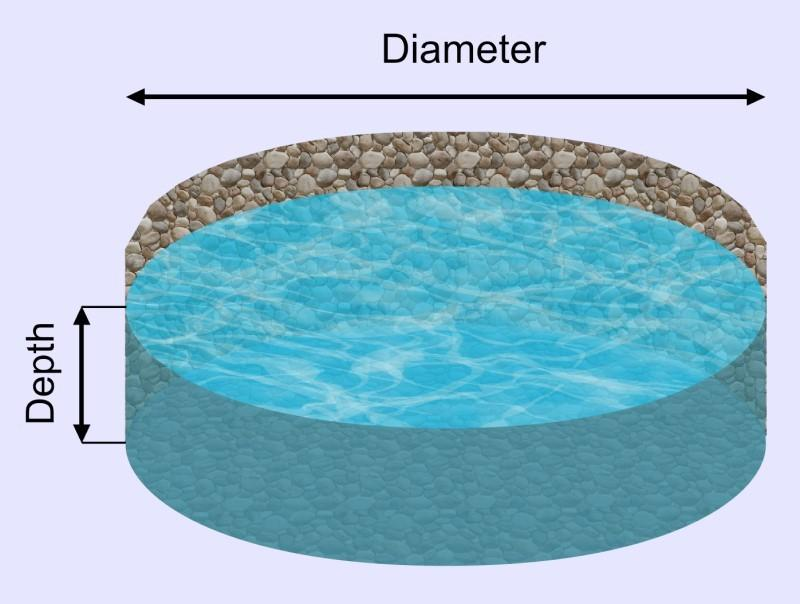For a circular pond, the surface area is calculated using the formula:

$$Surface\,Area= \pi × Radius^2$$

where

$$Radius = {Width \over 2}$$

and total pond volume is calculated by:

$$Volume = Surface\,Area × Depth$$

### Oval Pond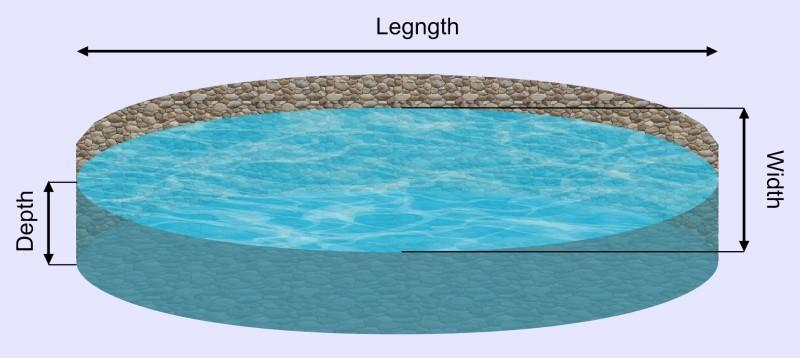For an oval pond, the surface area is calculated using the formula:

$$Surface\,Area= \pi × {Width \over 2} × {Length \over 2}$$

and total pond volume is calculated by:

$$Volume = Surface\,Area × Depth$$

Note that a circle is an oval with equal length and width!

## What are the units of volume?

The calculator gives answers for volume in liters, US or UK gallons, whereas the above calculations will give the answers in cubic feet.

Therefore, we need simply do the following conversions:

$$1\,ft^3 = 7.48\,US\,gallons$$

$$1\,ft^3 = 6.23\,UK\,gallons$$

$$1\,ft^3 = 28.32\,litres$$

Note that these are not exact conversions. However, the calculator does the exact conversions for you, so you don’t need to worry. 😉

## What if I’m using a pond liner?

A liner is a waterproof material that sits at the bottom of the pond. Its primary purpose is to ensure the pond remains watertight.

Imagine you have an irregular pond like this: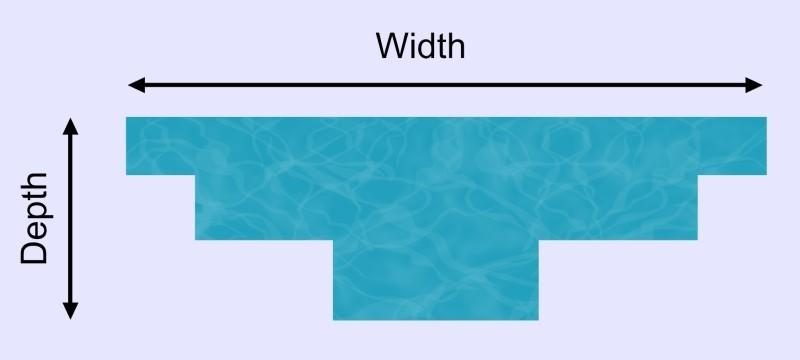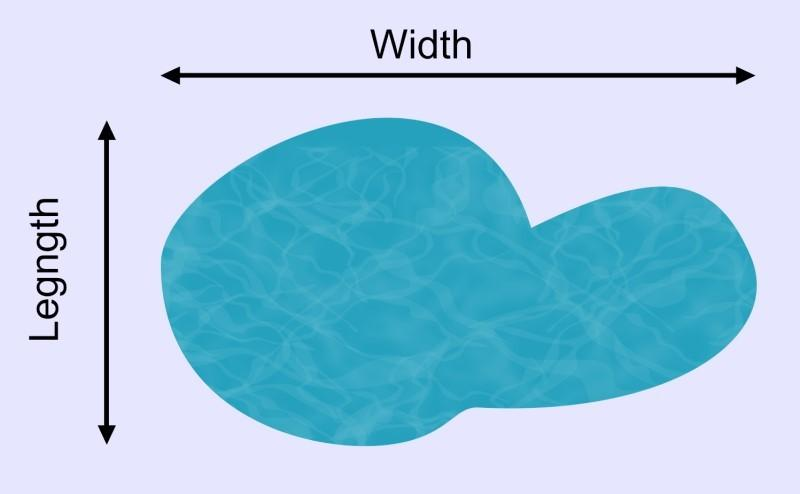We must allow for a “safety overlap” of 2 feet.

This helps us ensure that we have sufficient material to account for any mistakes in measuring etc.

The liner has dimensions:

$$Liner\,Length = Pond\,Length + Depth × 2 + 2\,feet$$

$$Liner\,Width = Pond\,Width + Depth × 2 + 2\,feet$$

We have to include the depth twice to account for the liner running from the top to the bottom of the pond and then from the bottom back to the top (i.e. twice).

Note: If you are using an irregular pond, then you should always use the maximum pond length and width in these calculations to ensure you have sufficient liner.

## Calculating the Pump Size

Ensuring you purchase a pump of sufficient size is crucial to your pond’s success.

It is recommended that you purchase a pump that circulates at least 50% of the ponds total volume every hour.

Therefore:

$$Minimum\,Pump\,Size = {Pond\,Volume \over 2}$$

Confused? 😕

See below for three fully worked examples where we calculate the surface area and volume of several ponds.

We will also show you how to calculate the liner size and recommend a minimum pump size for the given ponds.

Otherwise, simply enter your measurements into our online pond calculator!

## Example calculations

• Rectangular Fish Pond

Imagine my fish pond measures 10 feet in length, 12 feet in width and the bottom of the fish pond is at a constant depth of 5 feet.

I would enter these values into the pond calculator to work out the surface area:

$$Surface\,Area = Length × Width = 10\,ft × 12\,ft = 120\,ft^2$$

and the total volume of the fish pond:

$$Volume = Surface\,Area × Depth = 120\,ft^2 × 5\,ft = 600\,ft^3$$

If I want to calculate the volume in US gallons, I would use the conversion:

$$1\,ft^3 = 7.48\,US\,gallons$$

Therefore

$$600\,ft^3 = 4488.31\,US\,gallons$$

Now, to calculate the size of the liner required for the fish pond:

$$Liner\,Length = Pond\,Length + Depth × 2 + 2\,feet = 10 + 5 × 2 + 2 = 22\,ft$$

$$Liner\,Width = Pond\,Width + Depth × 2 + 2\,feet = 12 + 5 × 2 + 2 = 24\,ft$$

Finally, to calculate the recommended minimum pump size:

$$Minimum\,Pump\,Size = {Pond\,Volume \over 2} = {4488.3 \over 2} = 2244.15\,US\,gal/h$$

• Irregular Pond

Now, let’s imagine that we have an irregular pond shape.

The maximum width is 17 feet, and the maximum length is 12 feet.

Therefore, the surface area of the pond is:

$$Surface\,Area = Length × Width – 15\% = 17 × 12 – 15\% = 173.4\,ft^2$$

Note, to calculate a reduction of 15%, simply multiply by 0.85.

Now, let’s imagine the maximum depth of the pond is 10 feet.

The volume of the irregular pond can be calculated by:

$$Volume = {2 \over 3} × Length × Width × Depth = {2 \over 3} × 17\,ft × 12\,ft × 10\,ft = 1360\,ft^3$$

Let’s say that I want to calculate the volume in litres. Recall that:

$$1\,ft^3 = 28.32\,litres$$

Therefore:

$$1360\,ft^3 = 38510.9\,litres$$

Now, to calculate the size of the liner required for the pond is:

$$Liner\,Length = Pond\,Length + Depth × 2 + 2\,feet = 12 + 10 × 2 + 2 = 34\,ft$$

$$Liner\,Width = Pond\,Width + Depth × 2 + 2\,feet = 17 + 10 × 2 + 2 = 39\,ft$$

Finally, to calculate the recommended minimum pump size:

$$Minimum\,Pump\,Size = {Pond\,Volume \over 2} = {38510.9 \over 2} = 19255.5\,litres/h$$

• Oval Fish Pond

For our final example let’s imagine I want to know an estimate of how many US gallons of water it will take to fill an oval fish pond measuring 30 feet in width, 25 feet in length and 12 feet deep. The pond gallons calculator first figures out the surface area:

$$Surface\,Area= \pi × {Width \over 2} × {Length \over 2} = \pi × {30\,ft \over 2} × {25\,ft \over 2} = 589.049\,ft^2$$

and then the total volume of the fish pond:

$$Volume = Surface\,Area × Depth = 589.049\,ft^2 × 12\,ft = 7068.58\,ft^3$$

Then, to convert this into US gallons, recall that one cubic foot is equivalent to 7.48 US gallons. Therefore, we have:

$$7068.58\,ft^3 = 52876.68\,US\,Gallons$$

Now, to calculate the dimensions of the required total liner we must use the maximum length and width of the fish pond:

$$Liner\,Length = Pond\,Length + Depth × 2 + 2\,feet = 30 + 12 × 2 + 2 = 56\,ft$$

$$Liner\,Width = Pond\,Width + Depth × 2 + 2\,feet = 25 + 12 × 2 + 2 = 51\,ft$$

Be careful!

Don’t round your answers until the very last calculation to ensure accuracy throughout the calculations.

Finally,

## What to do if your units aren’t in feet

The online pond calculator does the conversions for you.

All you need to do is select the desired units in the drop down option and the calculator will do the following conversions for you:

$$1\,foot = 12\,inches = 0.33\,yards = 30.48\,centimeters = 0.3048\,meters$$

$$1\,ft^2 = 144\,in^2 = 0.111\,yd^2 = 929\,cm^2= 0.0929\,m^2$$

It couldn’t be simpler! 🙂

Embed this calculator on your site!Add live graphics
Copied to clipboard! Preview